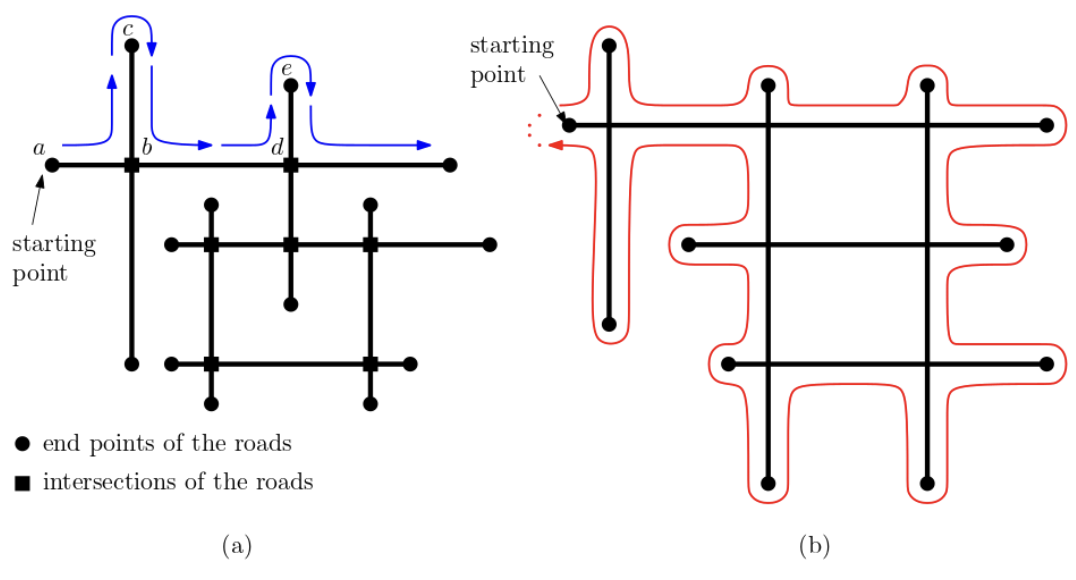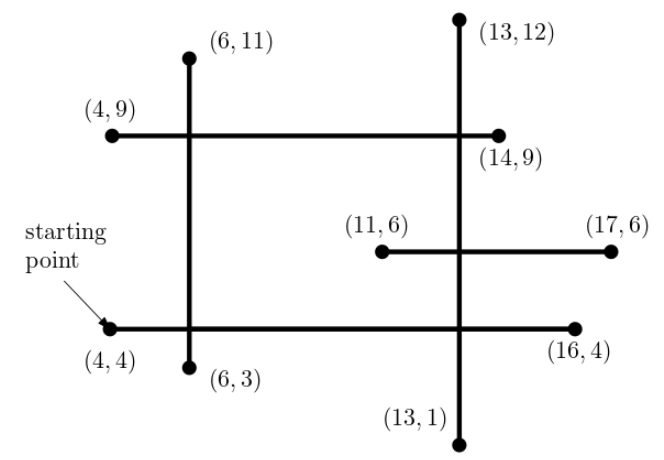시간 제한메모리 제한제출정답맞힌 사람정답 비율
1 초 512 MB205595333.333%

## 문제

One autonomous vehicle V is deployed in a mega factory with vertical and horizontal roads in the figure below. The roads are either horizontal or vertical segments. A vertical road and a horizontal road can meet at most once. If they intersect, then they intersect properly, that is, all the intersections are four-way. The end points of segments have integer coordinates.

The vehicle V starts at one end of some road at time t = 0 and moves at one unit per second according to a driving algorithm. V goes straight along the road until it meets either an intersection point of two roads or an end of the road. If it reaches the intersection, it turns left at that intersection. If it reaches the end of the road, it bounces back at that end and keeps moving according to the algorithm. The vehicle moves forever even when it comes back at the starting point. The time that V needs to take when it bounces back at the end of the road or turns left at the intersection point is negligible.

Let us explain with an example in Figure A.1(a). The starting point of the vehicle is the end point a. Then it first reaches the intersection b, turns left and moves toward the end point c. It bounces back at c and reaches b again. Then it turns left and moves along the part between b and d. It reaches another intersection d and turns left toward e, and so on. As in Figure A.1 (b), some parts of the segments are not traversed by the vehicle due to the turning rule of the algorithm, and the vehicle continues to move even when it comes back to the starting end point.

Given the roads of the factory, the starting end point of the vehicle V and a nonnegative time t, write a program to compute the coordinate (x, y) of the location of V at time t.Figure A.1 (a) The roads in the factory. (b) The vehicle comes back to the starting point.Figure A.2 The roads given in the second sample test case below.

## 입력

Your program is to read from standard input. The input starts with a line containing two integers, n (2 ≤ n ≤ 500) and t (0 ≤ t ≤ 109), where n is the number of roads, i.e., the number of line segments and t is the time at which you should output the location of the vehicle. In the following n lines, the i-th line contains four nonnegative integers bxi, byi, exi, eyi that represent two end points (bxi, byi) and (exi, eyi) of a (horizontal or vertical) segment. Two segments intersect at most one point. The starting end point for the vehicle is designated as (bx1, by1). All coordinates of the end points are between 0 and 107. No roads share the end points. Note that road segments are not necessarily connected and all the intersections are four-way.

## 출력

Your program is to write to standard output. Print two integers x and y representing the coordinate (x, y) of the vehicle at time t.

## 예제 입력 1

5 33
4 4 16 4
4 9 14 9
6 11 6 3
11 6 17 6
13 12 13 1


## 예제 출력 1

13 6


## 예제 입력 2

5 70
4 4 16 4
4 9 14 9
6 11 6 3
11 6 17 6
13 12 13 1


## 예제 출력 2

6 6